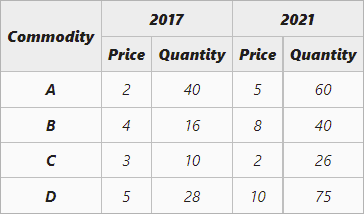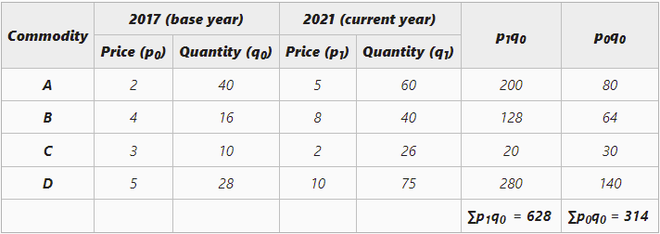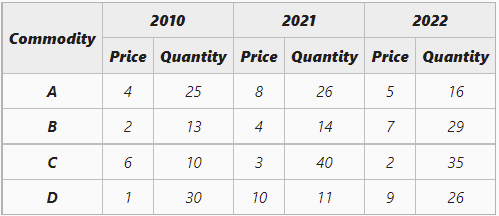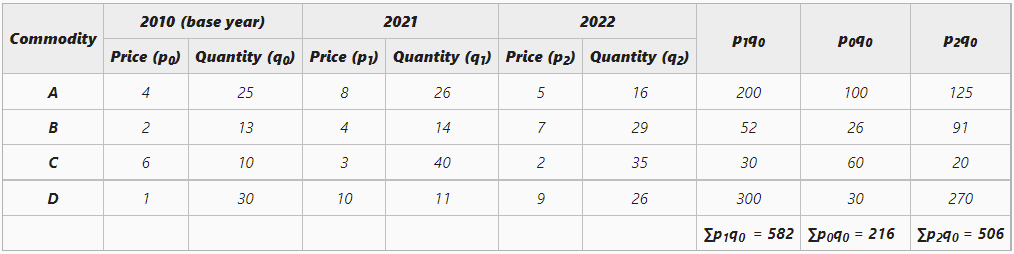GFG App
Open AppBrowser
Continue

# Laspeyre’s Method of calculating Weighted Index Number

A statistical measure that helps in finding out the percentage change in the values of different variables, such as the price of different goods, production of different goods, etc., over time is known as the Index Number. The percentage change is determined by taking a base year as a reference. This base year is the year of comparison. When an investigator studies different goods simultaneously, then the percentage change is considered as the average for all the goods. There are two broad categories of Index Numbers: viz., Simple and Weighted Index Numbers.

According to Spiegel, “An Index Number is a statistical measure designed to show changes in a variable or group of related variables with respect to time, geographic location or other characteristics.”

According to Croxton and Cowden, “Index Numbers are devices for measuring difference in the magnitude of a group of related variables.”

## Laspeyre’s Method

Weighted Index Numbers can be constructed by using two methods: Weighted Average of Price Relatives Method and Weighted Aggregative Method. Under the Weighted Aggregative Method, different weights are given to different goods according to the quantity bought. There are three methods through which weighted index numbers can be calculated under Weighted Aggregative Method, viz., Laspeyre’s, Paasche’s, and Fisher’s Method.

The method of calculating Weighted Index Numbers under which the base year quantities are used as weights of different items is known as Laspeyre’s Method. The formula for Laspeyre’s Price Index is:Here,

P01 = Price Index of the current year

p0 = Price of goods at base year

q0 = Quantity of goods at base year

p1 = Price of goods at the current year

#### Example 1

The following are the price and quantity of four commodities A, B, C, and D, in 2017 and 2021. Construct index number of prices in the year 2021 using Laspeyre’s Method.#### Solution:= 200

The Index Number of Price using Laspeyre’s Method is 200.

Example 2

From the data given below, construct Laspeyre’s Price Indices for 2021 and 2022 with the base year as 2010.#### Solution:Laspeyre’s Price Index for 2021:= 269.44

The price index for 2021 is 269.44

Laspeyre’s Price Index for 2022:= 234.25

The price index for 2022 is 234.25.

My Personal Notes arrow_drop_up